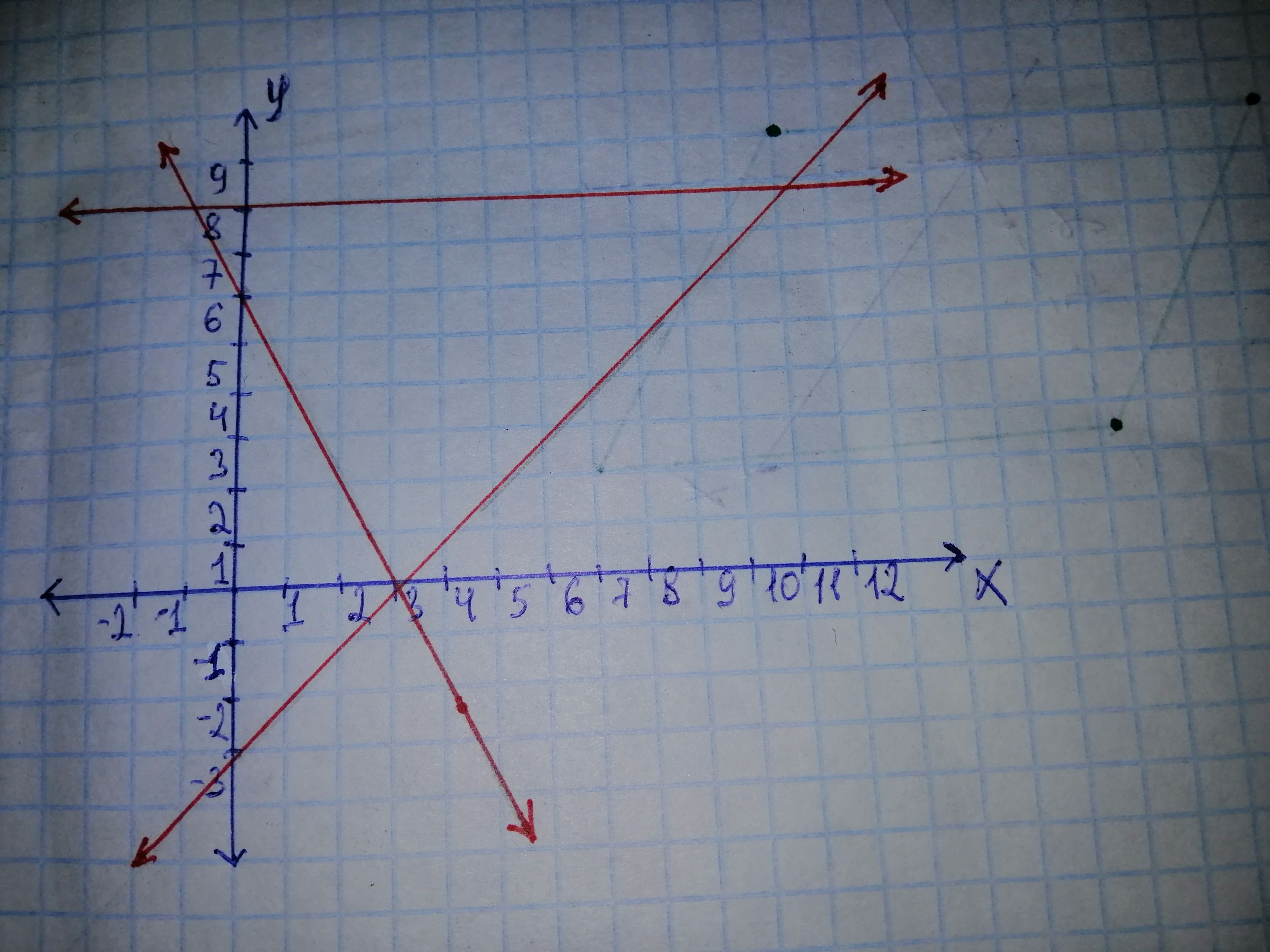# Use a graphing calculator to graph the solution of the system of inequalities. Find the coordinates of all vertices, rounded to one decimal place. begin{cases}y geq x - 3y geq -2x+6y leq 8end{cases}he298c 2020-11-08 Answered
Use a graphing calculator to graph the solution of the system of inequalities. Find the coordinates of all vertices, rounded to one decimal place.
$\left\{\begin{array}{l}y\ge x-3\\ y\ge -2x+6\\ y\le 8\end{array}$
You can still ask an expert for help

• Questions are typically answered in as fast as 30 minutes

Solve your problem for the price of one coffee

• Math expert for every subject
• Pay only if we can solve itWillie

Step 1
To draw graph for given inequality.Step 3
To find coordinate of all vertices
Plug
$8=x-3$
$x=8+3=11$
First coordinate is (11, 8)
Now plug
$8=-2x+6$
$-2x=2$
$x=-1$
second coordinate is (-1, 8)
Now solve system
equate both equation
$x-3=-2x+6$
$3x=9$
$x=3$
Plug
$y=3-3=0$
Third coordinate is (3, 0)
Therefore the coordinate of the vertex is